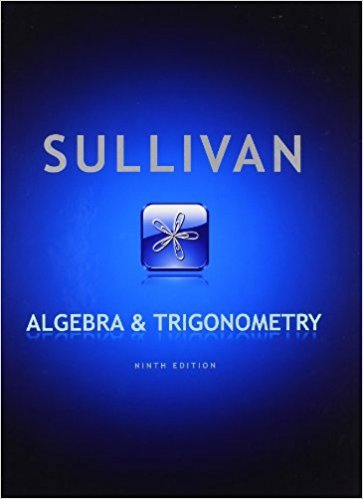×
×

# Solutions for Chapter 11.6: Algebra and Trigonometry 9th Edition## Full solutions for Algebra and Trigonometry | 9th Edition

ISBN: 9780321716569Solutions for Chapter 11.6

Solutions for Chapter 11.6
4 5 0 332 Reviews
23
1
##### ISBN: 9780321716569

This textbook survival guide was created for the textbook: Algebra and Trigonometry, edition: 9. Since 46 problems in chapter 11.6 have been answered, more than 58434 students have viewed full step-by-step solutions from this chapter. Algebra and Trigonometry was written by and is associated to the ISBN: 9780321716569. This expansive textbook survival guide covers the following chapters and their solutions. Chapter 11.6 includes 46 full step-by-step solutions.

Key Math Terms and definitions covered in this textbook
• Elimination.

A sequence of row operations that reduces A to an upper triangular U or to the reduced form R = rref(A). Then A = LU with multipliers eO in L, or P A = L U with row exchanges in P, or E A = R with an invertible E.

• Exponential eAt = I + At + (At)2 12! + ...

has derivative AeAt; eAt u(O) solves u' = Au.

• Gauss-Jordan method.

Invert A by row operations on [A I] to reach [I A-I].

• Hypercube matrix pl.

Row n + 1 counts corners, edges, faces, ... of a cube in Rn.

• Linearly dependent VI, ... , Vn.

A combination other than all Ci = 0 gives L Ci Vi = O.

• Nilpotent matrix N.

Some power of N is the zero matrix, N k = o. The only eigenvalue is A = 0 (repeated n times). Examples: triangular matrices with zero diagonal.

• Norm

IIA II. The ".e 2 norm" of A is the maximum ratio II Ax II/l1x II = O"max· Then II Ax II < IIAllllxll and IIABII < IIAIIIIBII and IIA + BII < IIAII + IIBII. Frobenius norm IIAII} = L La~. The.e 1 and.e oo norms are largest column and row sums of laij I.

• Normal matrix.

If N NT = NT N, then N has orthonormal (complex) eigenvectors.

• Orthonormal vectors q 1 , ... , q n·

Dot products are q T q j = 0 if i =1= j and q T q i = 1. The matrix Q with these orthonormal columns has Q T Q = I. If m = n then Q T = Q -1 and q 1 ' ... , q n is an orthonormal basis for Rn : every v = L (v T q j )q j •

• Particular solution x p.

Any solution to Ax = b; often x p has free variables = o.

• Pivot.

The diagonal entry (first nonzero) at the time when a row is used in elimination.

• Random matrix rand(n) or randn(n).

MATLAB creates a matrix with random entries, uniformly distributed on [0 1] for rand and standard normal distribution for randn.

• Rayleigh quotient q (x) = X T Ax I x T x for symmetric A: Amin < q (x) < Amax.

Those extremes are reached at the eigenvectors x for Amin(A) and Amax(A).

• Reflection matrix (Householder) Q = I -2uuT.

Unit vector u is reflected to Qu = -u. All x intheplanemirroruTx = o have Qx = x. Notice QT = Q-1 = Q.

• Schur complement S, D - C A -} B.

Appears in block elimination on [~ g ].

• Similar matrices A and B.

Every B = M-I AM has the same eigenvalues as A.

• Singular matrix A.

A square matrix that has no inverse: det(A) = o.

• Solvable system Ax = b.

The right side b is in the column space of A.

• Trace of A

= sum of diagonal entries = sum of eigenvalues of A. Tr AB = Tr BA.

• Wavelets Wjk(t).

Stretch and shift the time axis to create Wjk(t) = woo(2j t - k).

×